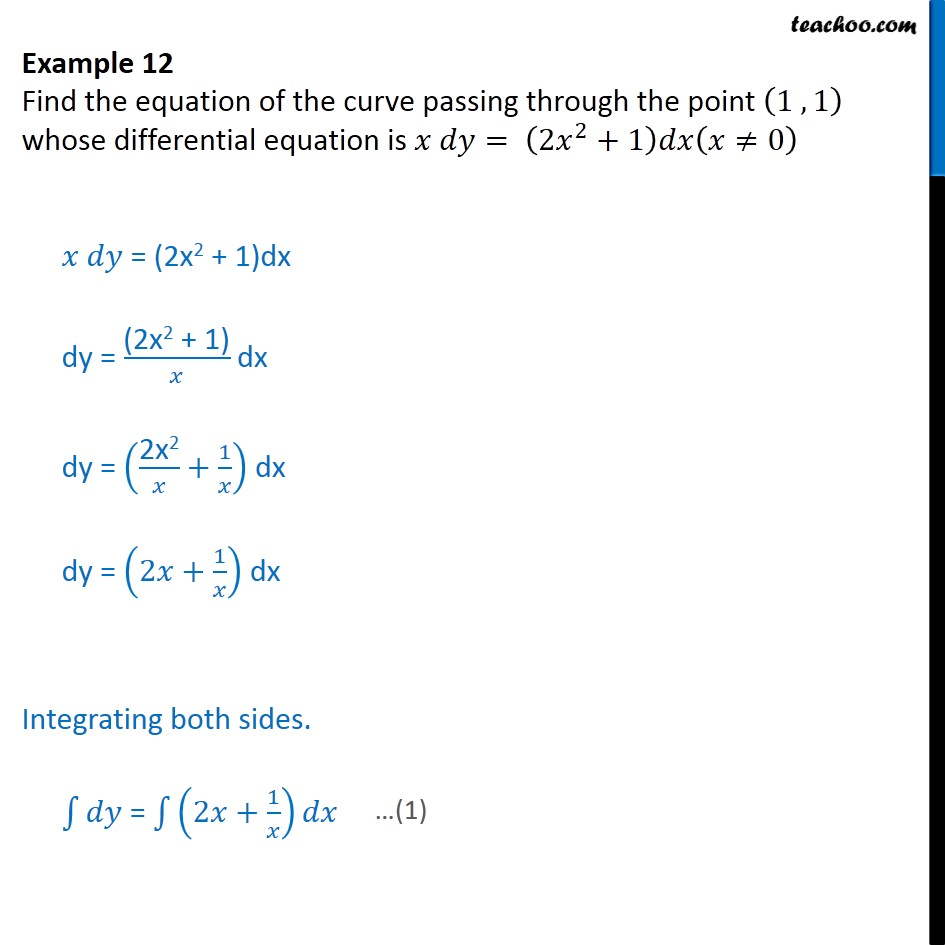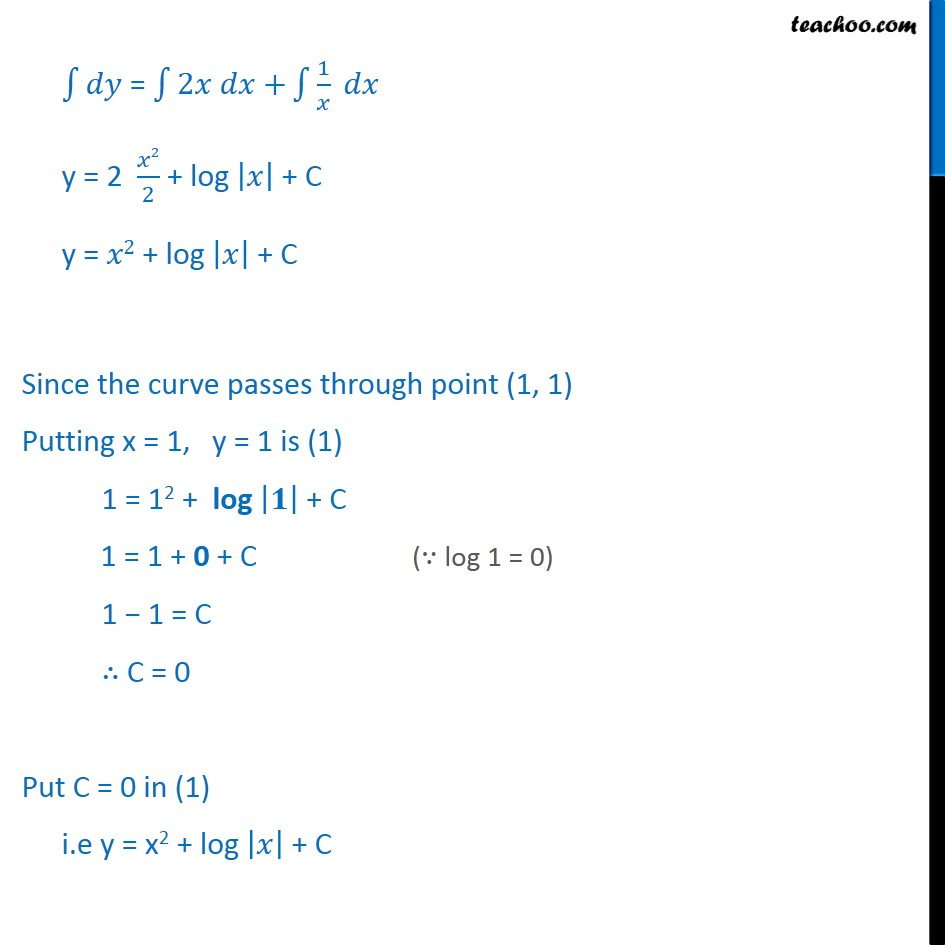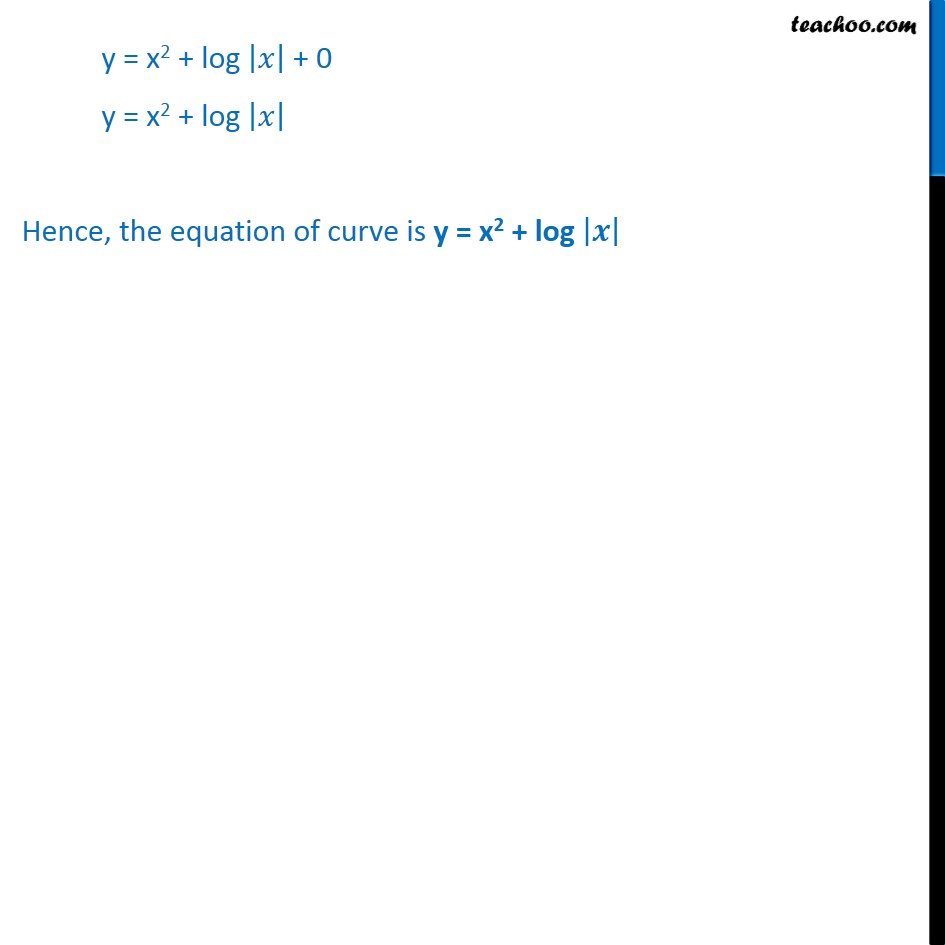Examples

Chapter 9 Class 12 Differential Equations
Serial order wiseLearn in your speed, with individual attention - Teachoo Maths 1-on-1 Class

### Transcript

Example 7 Find the equation of the curve passing through the point (1 , 1) whose differential equation is 𝑥 𝑑𝑦= (2𝑥^2+1)𝑑𝑥(𝑥≠0) 𝑥 𝑑𝑦 = (2x2 + 1)dx dy = "(2x2 + 1)" /𝑥 dx dy = ("2x2" /𝑥+1/𝑥) dx dy = (2𝑥+1/𝑥) dx Integrating both sides. ∫1▒𝑑𝑦 = ∫1▒(2𝑥+1/𝑥) 𝑑𝑥 ∫1▒𝑑𝑦 = ∫1▒〖2𝑥 𝑑𝑥+〗 ∫1▒〖1/𝑥 𝑑𝑥〗 y = 2 𝑥2/2 + log |𝑥| + C y = 𝑥2 + log |𝑥| + C Since the curve passes through point (1, 1) Putting x = 1, y = 1 is (1) 1 = 12 + log |𝟏| + C 1 = 1 + 0 + C 1 − 1 = C ∴ C = 0 Put C = 0 in (1) i.e y = x2 + log |𝑥| + C y = x2 + log |𝑥| + 0 y = x2 + log |𝑥| Hence, the equation of curve is y = x2 + log |𝒙|